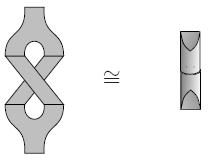# nLab Cardy condition

Contents

### Context

#### Functorial quantum field theory

functorial quantum field theory

# Contents

## Idea

The Cardy condition is part of the sewing constraint consistency condition on data that gives an open-closed 2d CFT or 2d TQFT.

It is the algebraic reflection of the fact that the following two cobordisms are equivalent (as topological as well as as conformal cobordisms):

1. an open string coming in, closing to a closed string and then opening up again to an open string (the “zip-unzip cobordism”);

2. an open string coming in, splitting into two open strings, these crossing each other (with the endpoints of one of them at the same time making a full rotation), then both merging again to one open string.For instance in the classification of open-closed 2d TQFT with coefficients in Vect via Frobenius algebras, this means that for $O$ the Frobenius algebra of open string states and for $C$ the commutative Frobenius algebra of closed string states, then the canonical linear function

$O \longrightarrow C \longrightarrow O$

is equal to the canonical map

$O \stackrel{\Delta}{\longrightarrow} O \otimes O \stackrel{\tau}{\longrightarrow} O \otimes O \stackrel{\mu}{\longrightarrow} O \,,$

where $\mu$ is the coproduct, $\mu$ the product and $\tau$ the braiding (e.g. Lauda-Pfeiffer 05 (2.14)).

Discussion in 2d TQFT includes

Discussion in 2d CFT includes